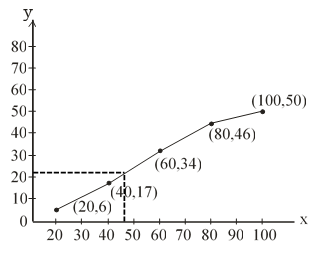#### 50 students enter for a school javelin throw competition. The distance (in metres) thrown are recorded below : Distance (in m) 0-20 20-40 40-60 60-80 80-100 Number of students 6 11 17 12 4 (i) Construct a cumulative frequency table. (ii) Draw a cumulative frequency curve (less than type) and calculate the median distance thrown by using this curve. (iii) Calculate the median distance by using the formula for median.(iv) Are the median distance calculated in (ii) and (iii) same ?(i) Solution. Frequency distribution: It tells how frequencies are distributed over values in a frequency distribution. However mostly we use frequency distribution to summarize categorical variables.

 Distance fi CF 0-20 6 6 20-40 11 6+11 = 17 40-60 17 17+17 = 34 60-80 12 34 + 12 = 46 80-100 4 46 + 4 =50

Solution. Frequency distribution: It tells how frequencies are distributed over values in a frequency distribution. However mostly we use frequency distribution to summarize categorical variables

 Distance Cumulative frequency (C.F) 0 less than 20 less than 40 less than 60 less than 80 less than 100 0 6 17 34 46 50

n = 50median = 49.41

Solution.  n = 50

which lies in interval 40 – 60
l
= 40, h = 20, CF = 17 and f = 17

=
=

=40 + 9.41
= 49.41

(iv) Yes, the median distance calculated in (ii) and (iii) are same.